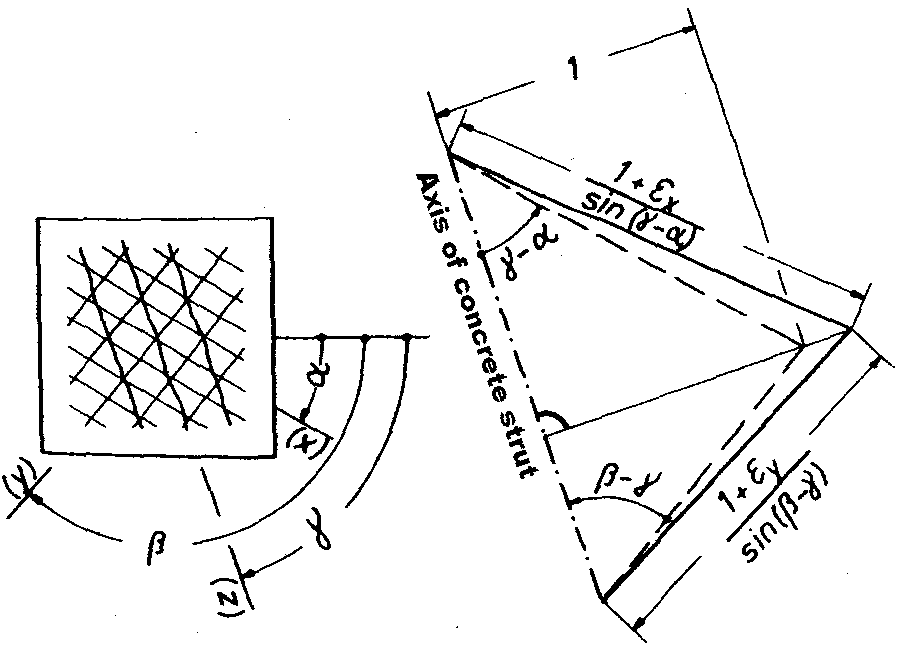# RF-CONCRETE Surfaces – Online Manual Version 5

Online manuals, introductory examples, tutorials, and other documentation.

# 2.6.1 Design Internal Forces

### Design Internal Forces

Unlike the transformation procedure for the ultimate limit state, it is not possible to carry out a purely geometrical division of the principal internal forces into internal forces in the individual reinforcement directions. Such a division assumes a strain ratio of 1.0 for the actual provided reinforcement. For both reinforcement directions to have the same strain, however, corresponding reinforcement areas would have to be respectively provided in these reinforcement directions for different design forces. In the serviceability limit state, however, the design internal forces are searched for a provided reinforcement.

In the serviceability limit state, no required reinforcement is determined; instead, the provided reinforcement is used to determine the actually provided strain ratio. In all cases where the applied reinforcement deviates from the required reinforcement, the actual provided strain ratio of the reinforcements does not equal the value 1.0.

The assumption of an identical strain ratio is therefore invalid. A different strain ratio that confirms the resulting design internal forces must be found. In solving this problem, the geometric relation between the strain ratio and the direction of the concrete compression strut plays an important role.

Baumann  writes the following on this point: If you neglect the compression strain of the concrete because it is usually small compared to the strain of the reinforcement, the following is obtained as the compatibility condition from Figure 38:

The following Figure 2.80 shows the mentioned Figure 38 with the compatibility condition of the strains for a two-directional reinforcement mesh.Figure 2.80 Compatibility of the strains

In Equation 2.62, εy and εx are the strains of two reinforcement directions. The angles α and β represent the intermediate angles between the principal force direction and the direction of the respective reinforcement set. The smaller intermediate angle is named α. The angle γ refers to the differential angle between the direction of the concrete compression strut and the direction of the first principal internal force.

The angles α and β cannot be changed due to the selection of the reinforcement direction. In contrast to this, the angle γ changes if a different direction of the concrete compression strut is necessary to stiffen the reinforcement mesh due to the varyingly stiff reinforcement directions.

The design internal forces in the individual reinforcement directions depend on the selected direction of the concrete compression strut. With these design internal forces, the stresses in the reinforcements of the individual directions can be determined. Based on these stresses, the various standards provide formulas with which you can determine the mean strains of the reinforcement relative to the concrete. In EN-1992-1-1, this is done according to Equation (7.9):

Only then can you determine the quotient from the differences in the strains between concrete and reinforcing steel of the second and first reinforcement direction.

Equation 2.62 also gives a quotient of the strains, derived from the geometric principles.

For both quotients, the strain of the second direction of reinforcement is in the numerator. This is based on the assumption that the first reinforcement direction forms the smaller differential angle with the first principal internal force. If the second reinforcement direction formed the smaller differential angle with the first principal internal force, the strains of the first reinforcement direction would be in the numerator of the quotient.

Both quotients depend on the selected direction of the concrete compression strut. The program now tries to select the direction of the concrete compression strut in such a way that both quotients become identical.

If the geometric strain ratio Qε,geo does not yet correspond to the actual strain ratio after one calculation run, the program specifies a new compression strut direction and determines the resulting geometric strain ratio. This process is repeated iteratively until a convergence is reached.

Determining the design internal forces by selecting the suitable compression strut direction is the most demanding part of the serviceability limit state design. If the selected provided reinforcement approximately corresponds to the statically required reinforcement for the analyzed service load magnitudes, the design internal forces only marginally differ from the internal forces that would result from an assumed strain ratio of 1.0. Therefore, RF-CONCRETE Surfaces additionally provides the possibility to determine design internal forces with an assumed strain ratio of 1.0.

Design internal forces for the serviceability limit state design are only determined if the cracking of the concrete leads to an activation of the reinforcement. To this end, the program analyzes the concrete tensile stresses caused by the first principal internal force.

Literatur
  Deutscher Ausschuss für Stahlbeton, Heft 217: Tragwirkung orthogonaler Bewehrungsnetze beliebiger Richtung in Flächentragwerken aus Stahlbeton (von Theodor Baumann). Verlag Ernst & Sohn, Berlin, 1972.